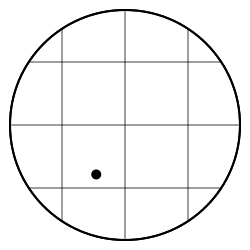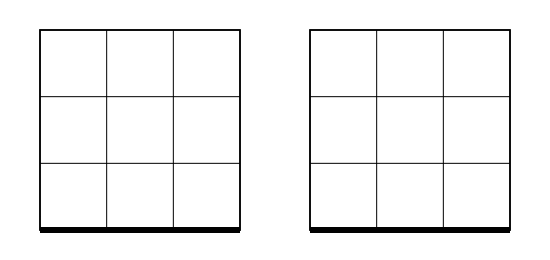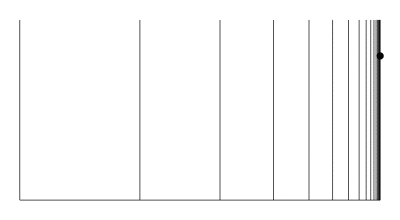# Deformation retraction

In mathematics (and topology in particular), a deformation retraction captures the idea of deforming a space over time into a subspace of itself.This figure illustrates an example of a deformation retraction of a disk into a subspace consisting of a single point.

Specifically, given a topological space $$X$$ and a subspace $$A$$ of $$X$$, a deformation retraction from $$X$$ to $$A$$ is a homotopy from the identity map on $$X$$ to a map from $$X$$ to $$A$$ that is the identity when restricted to $$A$$.

### Examples

For example, the first figure above illustrates a deformation retraction from a disk to one of its points.An annulus does not admit a deformation retraction to a point, like the one shown in bold. However, it does admit a deformation retraction to a core curve (also shown in bold).

In contrast, an annulus (like the one pictured on the right) does not admit a deformation retraction to a point. One way to see this is that a core curve, like the one in the interior shown in bold, is homotopically nontrivial, while a deformation retraction to a point would imply that the curve is homotopically trivial. An annulus, however, can easily be seen to admit a deformation retraction to a core curve.

## Strong deformation retraction

If the homotopy in the definition of a deformation retraction is the identity on $$A$$ for all maps in the family (so not just the first and final maps), then the deformation retraction is said to be a strong deformation retraction.

### Examples

The figure below shows an example illustrating the difference between a deformation retraction that's strong and one that isn't. The pictures on the left and right both show a deformation retraction from the square disk to the subspace defined by its bottom edge. The one on the left is strong because the homotopy preserves the subspace for all times. The one on the right isn't, however, because the edge subspace doesn't map to itself throughout the entire deformation.This figure shows what it means for a deformation retraction to be strong versus not strong. The left and right both depict deformation retractions from the square disk to its bottom edge. The one on the left is strong, while the one on the right isn't.

The topologist's comb, or comb space, provides another example. A typical topologist's comb is shown in the figure below. It consists of a horizontal segment along the bottom, a vertical segment along the right, and countably infinitely many vertical segments that approach (from the left) the vertical segment on the right.This figure depicts a (non-strong) deformation retraction from the topologist's comb to a point in its rightmost edge. No strong deformation retractions exist to points along this edge.

The topologist's comb is an example of a space with subspaces that admit a deformation retraction but no strong deformation retraction. An example of such a subspace is a subspace consisting of a single point in the rightmost segment, like the one shown in the figure in bold. The figure depicts an example of a non-strong deformation retraction for this subspace. As you can see, the deformation carries the point to the bottom edge before it returns to its original location, which is why it's not strong. However, no similar deformation retraction exists that's strong. (The reason a strong deformation retraction doesn't exist for points along the right edge is that the topologist's comb isn't locally connected at those points.)